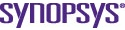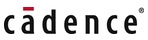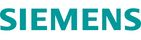# Efficient RC power grid verification using node elimination

By Ankit Goyal and Farid N. Najm, University of Toronto |  No Comments  |  Posted: June 2, 2011
Topics/Categories: EDA - Verification  |  Tags: , ,

To ensure the robustness of an integrated circuit, its power distribution network (PDN) must be validated beforehand against any voltage drop on VDD nets. However, due to the increasing size of PDNs, it is becoming difficult to verify them in a reasonable amount of time. Lately, much work has been done to develop Model Order Reduction (MOR) techniques to reduce the size of power grids but the focus is more on simulation. In verification, we are concerned about the safety of nodes, including the ones that have been eliminated in the reduction process. This paper proposes a novel approach to systematically reduce the power grid and accurately compute an upper bound on the voltage drops at power grid nodes that are retained. Furthermore, a criterion for the safety of nodes that are removed is established based on the safety of other nearby nodes and a user-specified margin. The work presented in this paper was supported in part by Advanced Micro Devices.

## 1. Introduction

The reliability of an integrated circuit design depends on its power distribution network (PDN). Any variations in supply voltage may lead to increased circuit delays and loss of yield. Thus, the voltage drop on any node of the grid must not exceed a certain threshold. This is crucial especially for modern designs that operate on reduced supply voltages.

One way to check the power grid integrity is through simulation. But it requires the knowledge of input currents used to load the grid, which might not be available, and an exhaustive analysis with all possible vector traces is impractical.

Another option is to do vectorless verification, which does not need the detailed information about the input stimuli. However, the techniques proposed in  and  require solving a linear program (LP) for every grid node.

Moreover, the size of each LP is proportional to the grid size. This imposes a limit on the size of grids that can be verified efficiently using such a vectorless approach. If we can reduce the grid size by eliminating some nodes, it will result in a reduced optimization problem with fewer and smaller LPs.

Model Order Reduction (MOR) gives a way to replace the original grid with a reduced one, with fewer nodes, while producing a good approximation of the input-output behavior.

MOR methods developed so far, such as those presented in  and , approximate the power grid by a smaller grid. When simulated, this grid has nearly the same output response as that of the original grid. These methods are not suitable for reducing a verification problem as they do not relate the safety of nodes in the original grid to those in the reduced grid. The key difficulty in our problem lies in characterizing the safety of nodes that have been eliminated during the reduction process.

Therefore, in the case of verification, besides maintaining electrical equivalence, the method must also be able to infer the safety of the original grid from the verification results of the reduced grid. In this work, we devise a safety criterion for the eliminated nodes based on worst-case voltage drops at the verified nodes in their proximity. Furthermore, the maximum voltage drop at nodes that are retained and verified is computed with minimal error.

## 2. Background

### 2.1 Power grid model

We consider an RC model of the power grid that has resistive connections between nodes and a capacitor from every node to ground. Some of the grid nodes have ideal current sources (to ground) representing the currents drawn by the circuits tied to the grid at those nodes. Further, some nodes have ideal voltage sources (to ground) that represent connections to the external supply voltage.

This configuration can be transformed into an equivalent grid model by removing all the voltage sources and reversing the direction of all current sources . The node voltages in this modified grid represents the voltage drops in the original network.

### 2.2 Vectorless verification

In order to capture the uncertainty about the circuit details and circuit behavior in early verification of the grid, we will use the concept of current constraints . There are two types of constraints, local constraints and global constraints. Local constraints are upper bounds on individual current sources and represent the maximum current drawn by individual cells or blocks. They can be represented as: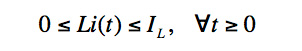where IL is a vector of fixed current values and L is an n X n identity matrix. If a grid node, k, does not have a current source attached to it, then IL,k = 0.

However, local constraints alone are insufficient, as the chip components do not draw their maximum currents simultaneously. Global constraints are introduced to provide an upper bound on the sum of currents drawn by groups of current sources, and can be expressed in matrix form as: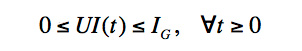where U is a matrix of dimension m × n such that Uij is 1 if the current source at node j is included in ith constraint, and 0 otherwise. Here, m is the number of global constraints, IG is a vector of size m and IG,i is the upper bound on the sum of currents included in the ith constraint.

## 3. Node safety criteria

A power grid node is considered safe if its voltage drop is less than a maximum allowable threshold value. In case of a purely resistive grid, the safety of a node is implied by the safety of all its immediate neighbors.

This comes from Kirchoff’s Current Law (KCL), according to which there must be at least one branch carrying current away from the node under consideration. Since this is a path of increasing voltage drop, at least one of the neighbors will have larger voltage drop than the given node. And therefore, the safety of all neighboring nodes implies the safety of a node.

However, in an RC power grid, a node can be connected to a capacitor and a current source besides resistive connections to other nodes. If somehow we can move these capacitors and sources to leave the node with resistive connections only, we no longer need to worry about its safety and it can be eliminated.

### 3.1 Moving current sources

The idea of moving a current source is not new and has appeared in research from time to time. However, this section explains the effect of moving a current source in the context of verification, with emphasis on its impact on node voltages and on the local and global constraints.

### 3.1.1 Prior work

Figure 1 shows a section of an RC power grid. We are interested in removing the current source at node 0. Note that the figure shows a node with four immediate neighbors but the analysis can easily be extended to any number of neighbors. The work in  describes how a current source present at a node can be moved to the neighboring nodes while preserving the electrical behavior of the power grid. The result is the introduction of a new current source at the immediate neighbor i of node 0, with a magnitude of: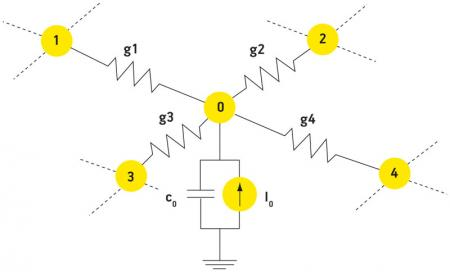Figure 1
A power grid section
Source: University of Toronto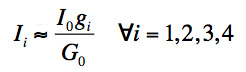where gi is the conductance between node 0 and node i, I0 represents the current source at node 0 and: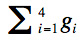Let c0 denote the capacitance to ground at node 0. The approximation involved in the above equation is based on quick node assumption , which requires c0/G0 to be less than τ. As we will see later on, only the nodes satisfying this property will be considered for elimination.

Although the electrical behavior of boundary nodes is preserved, node 0 voltage will change due to the removal of current source. Writing KCL at node 0 in the Laplace domain before and after moving the current source, and subtracting the two yields: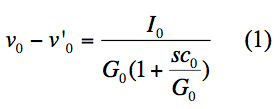where the two v values on the left of the equation are voltages at node 0 before and after moving the current source, respectively.

### 3.1.2 Application to verification

Because the values of the current sources are not known explicitly, they cannot be distributed directly as suggested above. Only the peak values of these sources are specified in terms of local and global constraints. Therefore, we have to express the above current source distribution scheme in terms of current constraints, in order to formulate the verification problem on a reduced grid.

### 3.1.2.1 Local current constraints

Let Ij be a feasible current assignment for some neighbor j of node i. Then, according to the local constraints, we have:The removal of the current source from node i will introduce a new current source at node j. Node j will now have this new current source in addition to any current source it may have had previously. Therefore, the modified feasible current assignment for node j, Ij, can be written as: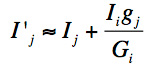where Ii is the feasible current assignment for node i, gj is the conductance connecting node i and node j and Gi is the sum of conductances connected to node i. From the above two equations, we have:Using IiIL,i in the above equation gives: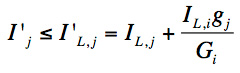where IL,j is the new value of local constraint at node j. Therefore, the modified local constraint is obtained by adding to its original value,  the local constraint value of its neighbor scaled by conductances. If we assume that there is no approximation involved, then the modified and the original system of constraints are equivalent and represent the same feasible space of voltages.

### 3.1.2.2 Global current constraints

Let there be m global constraints, represented as: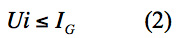If we remove a current source from node i, all entries in the ith column of U should then be set to zero. Besides this, if the kth constraint contains node i, then Ukj is set to 1 for every neighbor j of node i. Further, if neighbor j was not initially included in the kth constraint and has its own current source, then IG,k must be updated by adding to it the local constraint value corresponding to node j, IL,j. It can be easily seen that IG,k remains unchanged if the neighbor of node i has its own current source and is already included in constraint k. The modified global constraints may be represented as:Notice that the set of constraints in equations (2) and (3) are not equivalent. In fact, the feasibility space defined by (2) is a subset of that defined by (3). Consequently, in the absence of any approximations, the analysis performed using the modified global constraints guarantees a conservative estimate.

### 3.1.3 Implications for node safety

As noticed from (1), the movement of a current source from a node affects its voltage. Under quick node assumption, the first order Taylor expansion of this equation can be simplified to:Note that the voltage at node 0 is reduced in the modified network by a quantity approximately equal to I0/G0, referred to as the auxiliary voltage drop or simply the auxiliary drop of node 0. Therefore, whenever a current source is moved from a node, its auxiliary drop must be added to the node voltage in the modified network in order to obtain the actual node voltage.

### 3.2 Moving Capacitances

Consider again the generic node situation shown in Figure 1. After moving the current source, we want to move the capacitor from node 0 to its immediate neighbors. The target modified circuit will have capacitor ci added to neighbor i.Figure 1
A power grid section
Source: University of Toronto

### 3.2.1 Prior Work

The node elimination procedure in , distributes a capacitor among its neighbors in a weighted ratio of conductances. Analytically, this translates to: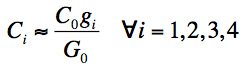Writing KCL in the Laplace domain at node 0 for the modified circuit and comparing it with the original gives: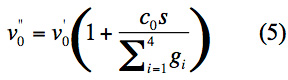where v’’0 is the new voltage at node 0.

### 3.2.2 Implications for node safety

Unlike the previous case of moving a current source, moving a capacitance away from a node will have negligible impact on its voltage. This is because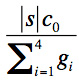is, by assumption, very small and utilizing this in equation (5) gives: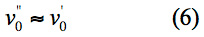This means that the voltage at node 0 in the modified network is approximately equal to that in the original network.

### 3.3 Characterizing Safety

After removing the current source and capacitor from node 0, it is connected to its neighboring nodes only via conductances. As noted here, the safety of all neighboring nodes would guarantee the safety of node 0. In terms of voltage drops: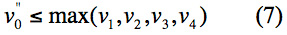Therefore, if all of the neighbors are known to be safe, node 0 must be safe as well. As we no longer need to directly verify node 0 for safety, it can be eliminated using an exact star-mesh transformation. The equivalent circuit shown in Figure 2 is obtained by removing node 0 and adding conductance gij between neighbors i and j, such that: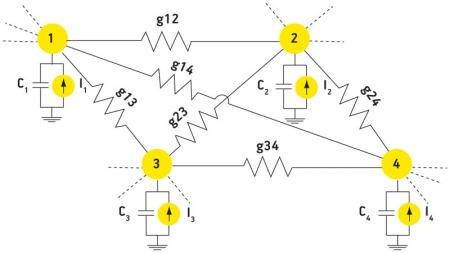Figure 2
Node elimination
Source: University of Toronto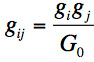where gi is the conductance between node 0 and neighbor i. However, besides ensuring the safety of the neighbors, we must also account for the auxiliary drop incurred due to current source removal. From Section 3.1.3, we know that removal of current source from node 0 results in an auxiliary drop of I0/G0. Also, recall that removal of a capacitor does not cause any additional auxiliary drop. Therefore, two factors define the safety of a node in the original grid: safety of its immediate neighbors and its auxiliary drop. From equations (4), (6) and (7), the criterion for safety of node 0 becomes: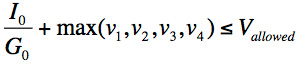where Vallowed is the maximum voltage drop allowed on any grid node.

## 4. Grid reduction

In the previous sections, we described a procedure to eliminate a node and establish its safety. We want to repeatedly apply the above node elimination procedure in order to eliminate whole sections of the grid, rather than a single node. However, the above procedure cannot be applied to all grid nodes, but only to the ones that have small c0/G0, as we have made this approximation at several instances.

Therefore, the first step will be to identify regions containing these special nodes. For doing this, the grid nodes are traversed topologically, starting from an arbitrary node, and all the adjacent nodes that have small c0/G0 are grouped together in the same region.

We must also keep track of nodes that will later be used to characterize the safety of nodes that have been removed. Define the safety set, X of a region to be the collection of all those nodes that will be included in the safety criteria of any internal (removed) node i in that region.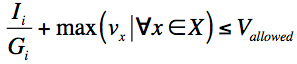As we expand the region, we keep updating the safety set by adding to it the neighbors of the node under consideration and removing from it, if present, the current node. As the region grows, the safety criteria may become pessimistic as a result of increased auxiliary drops and, possibly, due to maximization over a bigger set. Therefore, a user-specified limit on the size of regions is imposed. Once a region is created, its safety set must contain all its external boundary nodes.

These regions will initially include nodes having current sources as well as capacitors. Once we move these elements from internal nodes to the boundary, after accounting for auxiliary drops introduced, we would get regions having node-to-node conductances only. In case of such purely resistive regions, the voltage drop at any internal node must be less than some boundary node according to the same KCL argument given earlier. Therefore, the safety of an internal node, eliminated with star-mesh transformation, is governed by the safety of boundary nodes in addition to its auxiliary drop.

### 4.1 Computing Auxiliary Drops

We have seen how a current source can be moved from a node to its immediate neighbors. The result was the introduction of auxiliary drop at the node, along with secondary current sources at its neighbors. Because our aim is to move current sources all the way to the boundaries, the secondary sources created at the neighbors must be moved further away, one by one, until the boundary is encountered. The movement of secondary sources will introduce auxiliary drops at these neighbors as well.

Since the safety of a node is computed with respect to its neighbors, and if these neighbors themselves have auxiliary drops, then the auxiliary drop at the node must be updated by adding to it the maximum auxiliary drop among its neighbors. Moreover, as the exact values of current sources are not known, their maximum values specified in the local constraint vector, IL, are used to compute auxiliary drops.

We do not want these auxiliary drops to become too large because then the nodes may be falsely classified as unsafe. Therefore, if moving a current source causes the auxiliary drop at any node to exceed a user-specified threshold, γ, then the node containing that current source is excluded from the region and added to the safety set. Thus, the safety of nodes in the safety set will essentially guarantee the safety of all internal nodes within a margin, which is equal to the threshold value, γ itself.

Note that the number of secondary sources increases exponentially as we repeatedly move current sources. However, we can significantly reduce the complexity of this algorithm by pruning. The incremental auxiliary drops caused by a current source keep on decreasing as we move away from it. This is related to the concept of grid locality, which states that the effect of a current source diminishes as we move away from it . Practically, these drops become so small after a few hops away that there is no point in computing them. Hence, we can define a threshold, ε, on the auxiliary drops and prune everything beyond it, resulting in a near constant time algorithm.

### 4.2 Sparsity considerations

The star-mesh transformation results in the addition of new connections to each neighbor of the eliminated node. The total number of fill-ins created, across the whole grid, would be of the order of: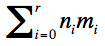where r is the total number of regions, ni is the number of internal nodes in the ith region, and mi is the number of boundary nodes of the ith region. A large number of fill-ins results in a denser constraint matrix, which may lead to slower optimization. This is another reason to limit the size of regions. This, in a sense, will reduce both ni and mi but with an increased r. Nevertheless, the reduction in the quadratic nimi is significant, compared to an increase in the linear effect of r.

Moreover, as we remove nodes from a region, many of the new connections added are insignificant in terms of magnitude and can be dropped without introducing much error. Therefore, in order to maintain sparsity, any new connections, which are below a certain threshold, κ, are dropped. This is similar to the approximation step of sparsification, which is often employed when working with large matrices. Another advantage of doing this is to avoid propagating insignificant connections as we remove nodes, thus making the reduction process faster.

## 5. Experimental results

A C++ implementation is written to evaluate the proposed approach. The grid verification problem is formulated as a linear program (LP) and solved using the upper-bound technique presented in . Our test grids were generated according to user specifications, which include grid dimensions, the number of metal layers, C4 and current source distributions, among others. The results are presented in Table 1 (p. 36) and are obtained for ε=0.05mV, γ=10mV, κ=1e−3 mho and τ=5e−12s. The size of a region is limited to 1% of total grid size in order to maintain sparsity. The percentage of nodes eliminated is shown in the second column. The CPU times for verifying the reduced and the original grids are shown. The runtime for the reduced grid includes the time to eliminate internal nodes and to compute auxiliary drops. Runtime for the last test case is estimated by verifying a thousand randomly chosen nodes and extrapolating the results.

For all the test cases, significant runtime saving is achieved. Furthermore, the maximum difference between the voltage drops in the reduced and original (retained) grid nodes is recorded and found to be negligible in all cases.

The safety of eliminated nodes can be established using the criteria developed earlier in this paper. Moreover, if all the verified nodes have voltage drops less than the allowed limit by an amount equal to γ, then the safety check on the eliminated nodes can be skipped and the grid may be considered safe.

## 6. Conclusion

With the growing size of power delivery networks, it is becoming difficult to verify them with traditional methods. The verification process is becoming increasingly costly in terms of time and computation resources, calling for an efficient model order reduction (MOR) technique.

However, existing MOR techniques are not suitable for verification. In this work, we have proposed a technique to selectively eliminate certain nodes of the power grid, while verifying the rest. The safety of the eliminated nodes is inferred from the safety of those that are verified within a predefined margin. Experimental results show excellent accuracy, along with significant runtime savings.

## 7. References

 D. Kouroussis and F. N. Najm, “A static pattern-independent technique for power grid voltage integrity verification”. Proc. 40th Design Automation Conference, pp99–104, June 2003.

 M. Nizam, F. N. Najm, and A. Devgan, “Power grid voltage integrity verification”. Proc. International Symposium on Low Power Electronics and Design, pp239–244, August 2005.

 B. Yan, S. X.-D.Tan, G. Chen, and L. Wu, “Modeling and simulation for on-chip power grid networks by locally dominant krylov subspace method”. Proc. International Conference on Computer-Aided Design, pp744–749, 2008.

 Y.-M. Lee, Y. Cao, T.-H. Chen, J.M. Wang, and C.C.-P. Chen, “HiPRIME: hierarchical and passivity preserved interconnect macromodeling engine for RLKC power delivery”. IEEE Transactions on Computer-Aided Design of Integrated Circuits and Systems, 24(6):797–806, June 2005.

 B. Sheehan, “Realizable reduction of RC networks”. IEEE Transactions on Computer-Aided Design of Integrated Circuits and Systems, 26(8):1393–1407, August 2007.

 A. J. van Genderen and N. P. van der Meijs, “Extracting simple but accurate RC models for VLSI interconnect”. Proc. International Symposium on Circuits and Systems, pp2351–2354, June 1988.

 E. Chiprout, “Fast flip-chip power grid analysis via locality and grid shells”. Proc. International Conference on Computer-Aided Design, pp485–488, November 2004.

 I. Ferzli, F. N. Najm, and L. Kruse, “A geometric approach for early power grid verification using current constraints”. Proc International Conference on Computer-Aided Design, pp40–47, November 2007.

Department of Electrical and Computer Engineering
University of Toronto
10 King’s College Rd
Toronto
Ontario